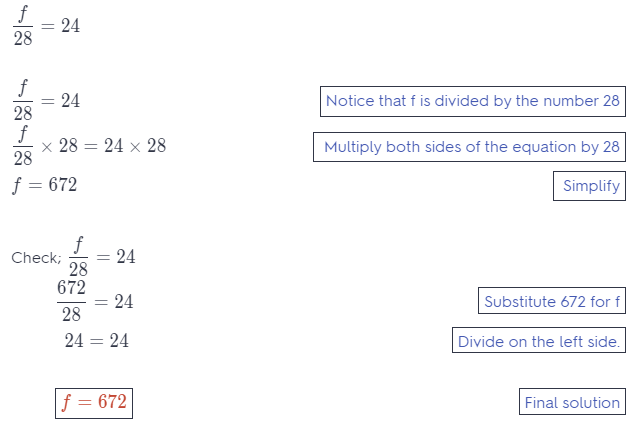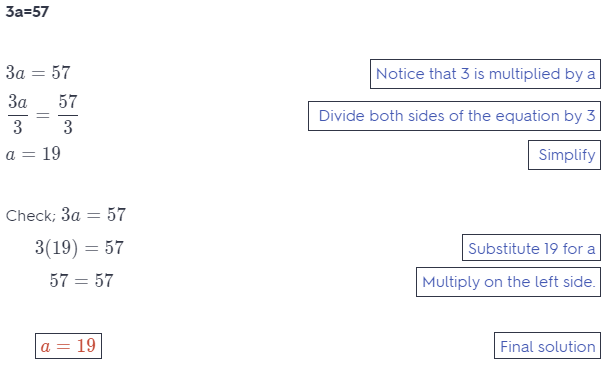Refer to our Texas Go Math Grade 6 Answer Key Pdf to score good marks in the exams. Test yourself by practicing the problems from Texas Go Math Grade 6 Module 12 Quiz Answer Key.

12.1 Writing Equations to Represent Situations

Determine whether the given value is a solution of the equation.

Question 1.
p – 6 = 19; p = 13
Solution or not
13 – 6 = 19 substitute for the value of p
7 ≠ 19    13 is not a solution
The given vaIue of 13 is not a solution to the equation.

Question 2.
62 + j = 74; j = 12.
Solution or not
62 – 12 = 74 substitute for the value of j
74 = 74     12 is a solution
The given value of 12 is a solution for the equation.

Question 3.
$$\frac{b}{12}$$ = 5; b = 60.
Solution to this example is given below
$$\frac{b}{12}$$ = 5; b = 60
$$\frac{60}{12}$$ = 5 Substitute 60 for b
5 = 5 Divide.
60 is a solution of $$\frac{b}{12}$$
b = 60

Question 4.
7w = 87; w = 12
Solution to this example is given below
7w = 87; w = 12
17(12) = 87 Substitute 12 for w
84 = 87 Multiply.
12 is a not of solution of the equation 7w = 87
w ≠ 12

Question 5.
18 – h = 13; h = -5.
Solution or not
18 – (-5) = 13 substitute for the value of h
18 + 5 = 13 add the numbers
23 ≠ 13 -5 is not a solution
The given value of -5 is not a solution for the equation.

Question 6.
6g = -86; g = -16
Solution or not
6 (-16) = -86 substitute for the value of g
-96 ≠ 86 -16 is not a solution
The given value of 16 is not a solution for the equation.

Write an equation to represent the situation.

Question 7.
The number of eggs in the refrigerator e decreased by 5 equals 18.
The total eggs were e and they decreased by 5 to reduced to 18, so the equation of the situation becomes: e – 5 = 18

Question 8.
The number of new photos p added to the 17 old photos equals 29.
Equation:
p + 17 = 29
where:
p is the number of new photos
17 is the number of old photos
29 is the total number of photos

Solve each equation.

Question 9.
r – 38 = 9
SoLution to this example is given below
r – 38 = 9
r – 38 + 38 = 9 + 38 Add 38 to both sides
r = 47 Simplify
r = 47

Question 10.
h + 17 = 40
Solution to this example is given below
h + 17 = 40
h + 17 – 17 = 40 – 17 Subtract 17 to both sides
h = 23 Simplify
h = 23

Question 11.
n + 75 = 155
Given equation:
n + 75 = 155
Add -75 on both sides of the given equation to isolate the variable on 1 side of the equation:
n + 75 – 75 = 155 – 75
Simplify to evaluate the variable:
n = 80

Question 12.
q – 17 = 18
Solution to this example is given below
q – 17 = 18
q – 17 + 17 = 18 + 17 Add 17 to both sides
q = 35 Simplify
q = 35

12.3 Multiplication and Division Equations

Solve each equation.

Question 13.
8z = 112
Given equation:
8z = 112
Divide both sides of the given equation with 8 to isoLate the variable on 1 side of the equation:
$$\frac{8z}{8}$$ = $$\frac{112}{8}$$
Simplify to evaluate the variable:
z = 14

Question 14.
$$\frac{d}{14}$$ = 7
Solution to this example is given belowQuestion 15.
$$\frac{f}{28}$$ = 24
Solution to this example is given belowQuestion 16.
3a = 57
Solution to this example is given belowEssential Question

Question 17.
How can you solve problems involving equations that contain addition, subtraction, multiplication, or division?
For explaining this question, lets consider an equation: 2x + 9 = 15 The first step to solve this equation will be to shift all the contents to the non variable side of the equation. This is done by adding 9 to both sides of the equation so the equation transforms to 2x = 15 – 9 = 6. The next step to change the coefficient of x to 1. This is done by dividing both sides of the equation with 2, so the equation transforms to x = $$\frac{6}{2}$$ = 3. This is the solution of the equation.

Texas Go Math Grade 6 Module 12 Mixed Review Texas Test Prep Answer Key

Selected Response

Question 1.
Kate has gone up to the chalkboard to do math problems 5 more times than Andre. Kate has gone up 11 times. Which equation represents this situation?
(A) a – 11 = 5
(B) 5a = 11
(C) a – 5 = 11
(D) a + 5 = 11
(D) a + 5 = 11

Explanation:
Let Andre’s turns be a and Kate has had 5 more turns so the equation to represent the number of Kates turns becomes: a + 5 = 11.

Question 2.
For which equation is y = 7 a solution?
(A) 7y = 1
(B) y – 26 = -19
(C) y + 7 = 0
(D) $$\frac{y}{2}$$ = 14
(B) y – 26 = -19

Explanation:
(A) 7 – (7) = 1 substitute for the value of y
49 ≠ 17 is not a solution

(B) 7 – 26 = – 9 substitute for the value of y
-19 = -19 7 is a solution

(C) 7 + 7 = 0 substitute for the value of y
14 ≠ 0 7 is not a solution

(D) $$\frac{7}{2}$$ = 14 substitute for the value of y
3.5 ≠ 14 7 is not a solution

The equation (B) y – 26 = -19 shows that the value of 7 is a solution.

Question 3.
Which is an equation?
(A) 17 + x
(B) 45 ÷ x
(C) 20x = 200
(D) 90 – x
(C) 20x = 200

Explanation:
An equation is presented by 2 different expressions equated with each other by an equal to sign, therefore option C shows an equation.

Question 4.
The number line below represents which equation?(A) -4 + 7 = 3
(B) -4 – 7 = 3
(C) 3 + 7 = -4
(D) 3 – 7 = -4
(D) 3 – 7 = -4

Explanation:
The arrow started at 3 and moved to the left 7 gaps and ended at -4. Therefore the equation for the number line is 3 – 7 = -4.

Question 5.
Becca hit 7 more home runs than Beverly. Becca hit 21 home runs. How many home runs did Beverly hit?
(A) 3
(B) 14
(C) 21
(D) 28
(B) 14

Explanation:
Let Beverly runs be a and Becca has hit 7 more runs so the equation to represent Becca’s runs is: x + 7 = 21. Solve this equation for x, therefore: x = 21 – 7 = 14.

Question 6.
Jeordie spreads out a rectangular picnic blanket with an area of 42 square feet. Its width is 6 feet. Which equation could you use to find its length?
(A) 6x = 42
(B) 42 – x = 6
(C) $$\frac{6}{x}$$ = 42
(D) 6 + x = 42
(A) 6x = 42

Explanation:
The area of a rectangular is the product of its length and width Therefore, 6x = 42 is the equation to be used to evaluate x, the length of the rectangular blanket.

Question 7.
What is a solution to the equation 6t = 114?
(A) t = 19
(B) t = 108
(C) t = 120
(D) t = 684
(A) t = 19

Explanation:
Examine each part of the equation.
t is the unknown value you want to find.
6 is multiplied by t.
= 114 means that after multiplying 6 and t, the result is 114.
Use the equation to solve the problem.
6t = 114
$$\frac{6 t}{6}=\frac{114}{6}$$ Divide both sides by 6
t = 19 Simplify
A is the option is correct answer.

Question 8.
The area of a rectangular deck is 680 square feet. The deck’s width is 17 feet. What is its length?
(A) 17 feet
(B) 20 feet
(C) 40 feet
(D) 51 feet
(C) 40 feet

Explanation:
A = l ∙ w formula for the area of a rectangle
680 square feet = l ∙ 17 feet substitute for the given values
$$\frac{680}{17}=\frac{l \cdot 17}{17}$$ divide both sides of the equation by 17
40 feet = l length of the rectangle
The length of the rectangular deck is 40 feet.

Gridded Response

Question 9.
Sylvia earns $7 per hour at her after school job. One week she worked several hours and received a paycheck for$91. Write and solve an equation to find the number of hours in which Sylvia would earn \$91.$$\frac{7 x}{7}=\frac{91}{7}$$ divide both sides of the equation by 7